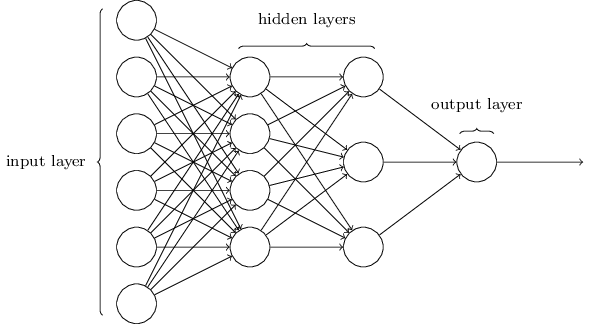# Chansh

## Keep on writing.

### 一、深度网络架构第一层和最后一层分别代表输入层和输出层，中间的都叫隐层。一般来说只有前后两层中间有连线，不过也有一些网络是跨层或者同层间相连的。因为是入门，所以这里只讨论最基础的结构。

\begin{eqnarray} z^l&=&w^l a^{l-1}+b^l,
a^l&=&\sigma(z^l). \end{eqnarray}

$a^l$是l层中所有节点的输出值的向量，$w^l$是l-1层中各个节点到l层中各个节点的权值的向量，$b_j^l$是l层各个节点计算时的偏量的向量。函数$\sigma$激活函数，经常使用的有sigmoid、tanh、softmax、ReLu等。1

$y(x)$是样本的label，$a^L(x)$是输出层输出的结果。$C$越小，则训练效果越好。

$\eta$是下降的步长。记

Written with StackEdit.

1. 图片来源于维基百科Sigmoid